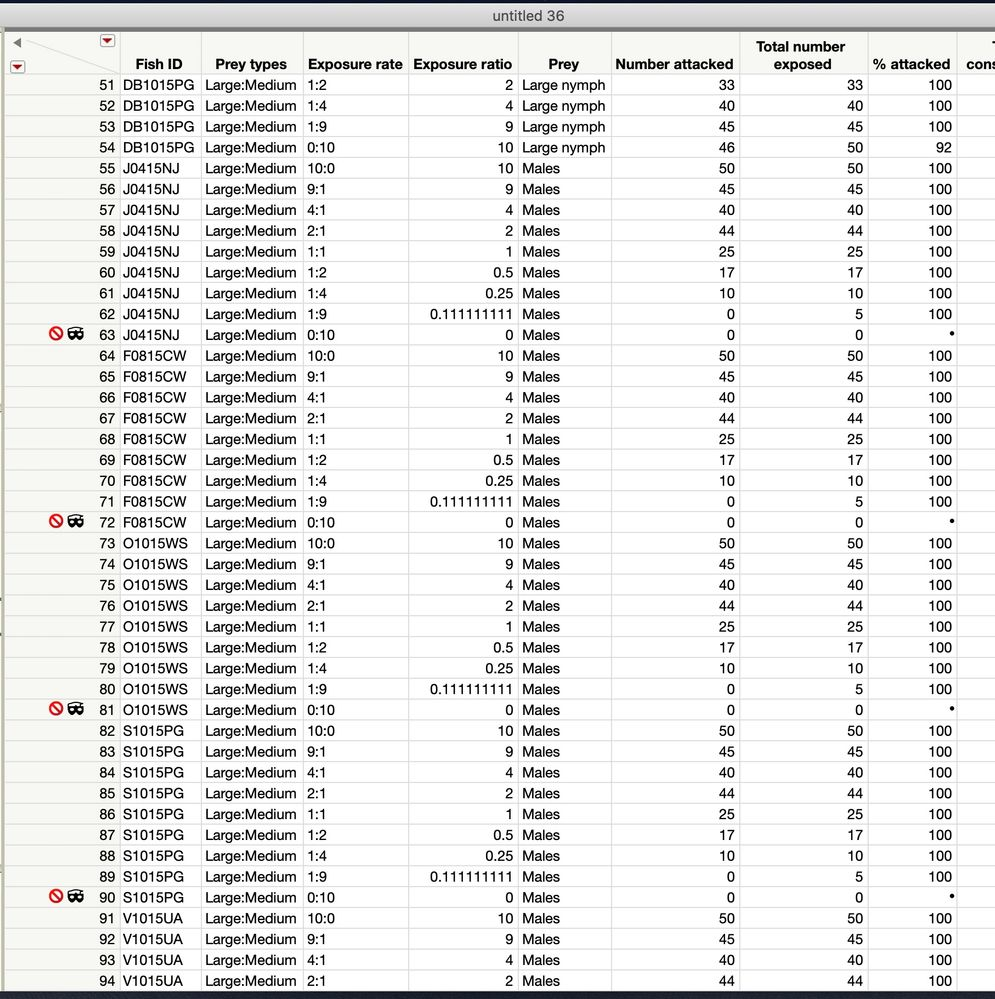Choose Language Hide Translation Bar
Highlighted

## Beta Binomial Generalized Regress Variables

I have a dataset that is constructed like this:

 ID Replicate Y X1 X2 A 1 0 .78 .33 A 2 0.25 .44 .09 B 1 0.25 .76 .66 B 2 1 .31 .39 C 1 0 .11 .48 C 2 0 .07 .22

The beta binomail distribution seems appropriate because my response variable (Y) is porportional.  Thus, I am interested in looking at the relationship of the two X variables to Y.  In order to use a beta binomial regression in jmp, you need to:

The beta binomial distribution requires a sample size greater than one for each observation. Thus, the user must specify a sample size column. To insert a sample size column, specify two continuous columns as Y in this order: the count of the number of successes, and the count of the number of trials. The link function for p is the logit.

Has anyone ever used this type of analysis and understands how to arrange the dataset to perform it correctly?  Thank you for your help!

3 REPLIES 3
Highlighted

## Re: Beta Binomial Generalized Regress Variables

Yes, JMP requires two columns in the Y role. The order of entry in the Y role is important. The first data column is a count of successes (as you define success) and the second column is the count of the total observed (successes plus failures). So, for example, you might have observed 10 cases but none of them were successes when ID=A and Replicate=1. Instead of a proportion of 0, enter a count of 0 and a size of 10. You need to replace the proportional Y in your data table with two data columns.

Learn it once, use it forever!
Highlighted

## Re: Beta Binomial Generalized Regress Variables

Thank you for your help!  That is much more clear!

Highlighted

## Re: Beta Binomial Generalized Regress Variables

I'm fitting a beta binomial generalized regression but the regression plot in the output is not accurate.   The number of success (or number attacked) should be between 0 and 50 but in the plot is never above 5. Attached is a screenshot of the data and output.  Any ideas what is going on here?Article Labels

There are no labels assigned to this post.